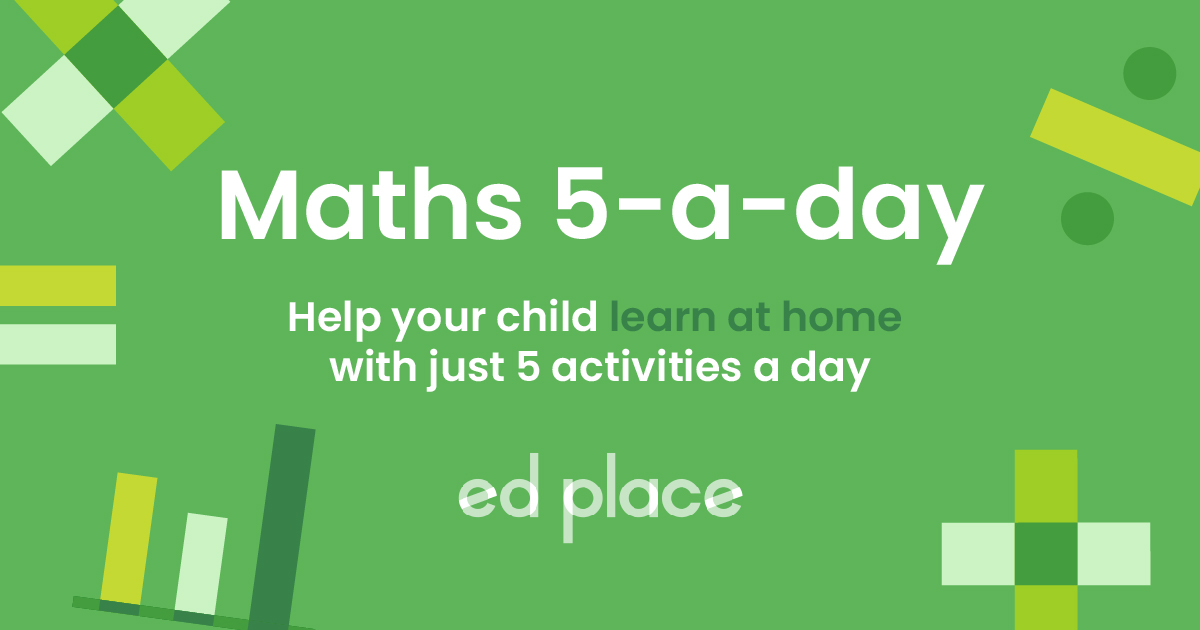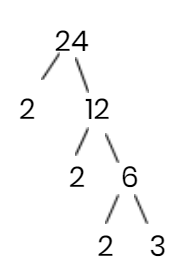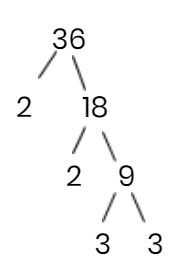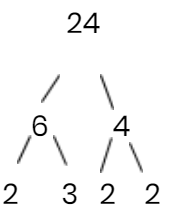# EdPlace's GCSE Home Learning Maths Lesson: Highest Common Factors

Looking for short lessons to keep your child engaged and learning? Our experienced team of teachers have created English, maths and science lessons for the home, so your child can learn no matter where they are.  And, as all activities are self-marked, you really can encourage your child to be an independent learner.

Get them started on the lesson below and then jump into our teacher-created activities to practice what they've learnt. We've recommended five to ensure they feel secure in their knowledge - 5-a-day helps keeps the learning loss at bay (or so we think!).

Are they keen to start practising straight away? Head to the bottom of the page to find the activities.

Now...onto the lesson!

GCSE Specification Requirements
GCSE students should be able to use the ideas and vocabulary of prime numbers, factors, common factors, highest common factors and prime factorisation.

# Can Your Child Successfully Identify the Highest Common Factor?

Your child has come to you and said ‘What is the highest common factor? I need your help.’ You can feel the stress level starting to rise and it's yours. Smiling you say to your eager child, ‘of course I can help’. Your brain is starting to whir and think, where have I heard this before? The chances are, probably not since you sat your own maths examinations way back when! Help is at hand. Here at EdPlace, we’re surrounded by a team of experts who communicate many concepts with children on a day-to-day basis, and we’re ready to share their teaching gems with you.

We're confident that if you follow the step-by-step approach below your child will be able to:

1) Understand the key terms from this topic

2) Apply their knowledge to confidently identify the highest common factor

3) Explain the reasoning behind their answer

## Step 1 - Key Terminology

To get started we need to know the definition of a few keywords. It's important to check that your child understands what some key terminology means as they pop up regularly in their lessons, textbooks and of course, exam papers.

Factor: A number that divides equally into another number. For example, the factors of 8 are 1, 2, 4 and 8. The factors of  24 are 1, 2, 3, 4, 6, 8, 12 and 24. The factor of 1 is 1 (this is important to remember when it comes to prime numbers). As you can see some numbers have more factors than others.

Prime Number: is a number that has only two factors, that is the number one and itself. For example, the factors of 3 are 1 and 3. The factors of 5 are 1 and 5. The factors of 7 are 1 and 7.

Beware! A common mistake is that students often think that all odd numbers are prime. Let’s just look at that for a minute. 1 is not prime, it only has one factor (see above). 9 is not prime, it's factors are 1, 3 and 9. Remember the rule of only two factors.

Top Tip: There are no even prime numbers except for 2 as it's the only one to fit the prime number rule. If you're unsure whether a number is prime of not, just try and divide it by 3 and 7. If both numbers cannot divide into it equally then it is prime.

Common factor: It's basically what it says on the tin. Your child needs to break down numbers and look for values that are the same...

## Step 2 - How to Find the Highest Common Factor

To find the highest common factor (HCF) it's necessary to break down two numbers into their product of prime numbers. This just means to divide the number until you are left with a string of prime numbers. If your child struggles to remember the first few prime numbers, you can tell them the following joke: ‘Oh no, 4, 6, 8 and 9 have been kidnapped. The prime suspects are 2, 3, 5, 7, 11.'

## Step 3 - Diagrams

What is the highest common factor of 24 and 36?

Many times a child will think that they have to hold all the information in their head until they have an answer. However, this is a sure way for your head to explode before you've even started. It's important that your child takes one step at a time. Additionally, clear workings out are a sure-fire way to pick up extra marks in an exam even if your final answer is wrong.

Diagrams help us get the information down and marks awarded. Let’s start with breaking down 24 into prime numbers. What numbers can you think of that multiple together to get 24? There is a prime number here so we put a circle around it and leave it alone. We now repeat the process for the number 12, and then against for 6. As you can see in the diagram below, 24 has been broken down into its product of prime numbers.Product means to multiply, to check you're correct just multiply the prime numbers together: 2 x 2 x 2 x 3 = 24.

Repeat this process for 36. What numbers can you think of that multiply together to get 36? There is a prime number here so we put a circle around it and leave it alone. We now repeat the process for the number 18, and then again for 9.Check: 2 x 2 x 3 x 3 = 36.

NoteYou may not always break the number down by 2. For example, you could have started breaking down 24 as follows.As you can see you will arrive at the same answer.

## Step 4 - Put Your Knowledge to the Test...

Let’s use what we've revised to find the highest common factor.

Prime factors of 24 = 2, 2, 2, 3.

Prime factors of 36 = 2, 2, 3, 3.

You are now going to look for pairs of numbers to use. Look at one set of numbers and find its pair in the other set of numbers. Don’t worry about the numbers not paired. These will give you a great little bonus later.

Prime factors of 24 = 2, 2, 2, 3. Highest Common Factor 2 x 2 x 3 = 12.

Prime factors of 36 = 2, 2, 3, 3.

The highest common factor is the biggest number that will divide into both 24 and 36. Quite often your child will see this straight away and say ‘what was the point of all that writing? My hand hurts now’. Those pesky examiners want to know how your child knows in order to award the highest marks.

Bonus challenge: quite often your child may be asked to find the lowest common multiple. Once your child has found the highest common factor they can use that number, which in our example was 12, and multiply it with the numbers that weren’t paired up. So, the lowest common multiple is 12 x 2 x 3 = 72. This means that if you wrote out the 24 times table and 36 times table the first number that appears in both is 72.

Practice Questions:

1) How can you tell if a number is prime?

2) What even number(s) are prime?

3) What is a factor?

4) Find the Highest Common Factor of  28 and 14.

5) Find the Highest Common Factor of 60 and 84.

## Step 5 - Over to you

Now that you’ve learnt to identify the highest common factor and lowest common multiple, see if your child can apply their knowledge to the following 5 activities. Have them complete them in the order listed below. All activities are created by teachers and automatically marked.

Plus, with an EdPlace subscription, we can automatically progress your child at a level that's right for them. Sending you progress reports along the way so you can track and measure progress, together - brilliant!

1) A prime number has only two numbers (factors) that divide into it. The number 1 and itself.

2) Only the number 2.

3) A factor is a number that divides equally into another number.

4) 14

5) 12

6) 84

Keep going! Looking for more activities, different subjects or year groups?

Click the button below to view the EdPlace English, maths, science and 11+ activity library